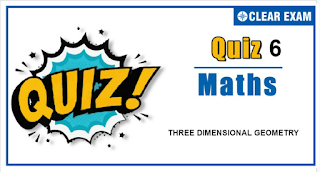## Three Dimensional Geometry Quiz

Important topics for Maths has been designed in such a way that it offers very practical and application-based learning to further make it easier for students to understand every concept or topic by correlating it with the day-to-day experiences.

Q1. The shortest distance between the lines (x-3)/3=(y-8)/(-1)=(z-3)/1 and (x+3)/(-3)=(y+7)/2=(z–6)/4 is
•  √30
•  2√30
•  5√30
•  3√30
Solution
(d) Given lines are r =3i ̂+8j ̂+3k ̂+l(3i ̂-j ̂+k ̂) and r =-3i ̂-7j ̂+6k ̂+m(-3i ̂+2j ̂+4k ̂) Required shortest distance =(|(6i ̂+15j ̂-3k ̂ ).((3i ̂-j ̂+k ̂)×@(-3i ̂+2j ̂+4k ̂))|)/(|(3i ̂-j ̂+k ̂)×(-3i ̂+2j ̂+4k ̂)|) =(|(6i ̂+15j ̂-3k ̂ ).(-6i ̂-15j ̂+3k ̂ )|)/(|(-6i ̂-15j ̂+3k ̂)|) =(36+225+9)/√(36+225+9)=270/√270=√270=3√30

Q2.If a line makes an angle of π/4 with the positive direction of each of x-axis and y-axis, then the angle that the line makes with the positive direction of the z-axis is
•  π/3
•  π/4
•  π/2
•  π/6
Solution
(c) Here l=cos π/4,m=cos⁡π/4 Let the line make an angle ‘γ’ with z-axis ∴l^2+m^2+n^2=1 ⇒cos^2⁡π/4+cos^2⁡π/4+cos^2⁡γ=1 ⇒1/2+1/2+cos^2⁡γ=1 ⇒2 cos^2⁡γ=0 ⇒cos⁡γ=0 ⇒ γ=π/2

Q3.  The length of projection of the line segment joining the points (1,0,-1) and (-1,2,2) on the plane x+3y-5z=6, is equal to
•   2
•  √(271/53)
•  √(472/31)
•  √(474/35)
Solution
(d) Let A(1,0,-1),B(-1;2,2) Direction ratios of segment AB are <2,-2,-3> cos⁡θ=|2×1+3(-2)-5(-3)|/(√(1+9+25) √(4+4+9))=11/(√17 √35)=11/√595 Length of projection =(AB) sin⁡θ =√((2)^2+(2)^2+(3)^2 )×√(1-121/595) =√17 √474/(√17 √35)=√(474/35) units

Q4. Distance of point P(p ⃗) from the plane r ∙n =0 is
•  |p ∙n |
•  (|p×n|)/(|n|)
•  (|p∙n|)/(|n|)
•  None of these
Solution
(c) Let Q(q) be the foot of altitude drawn from ‘P’ to the plane r∙n=0 ⇒q-p=λn ⇒q=p+λn Also q∙n=0⇒ (p+λn)∙n=0 ⇒λ=-(p∙n)/|n|^2 ⇒q -p =-((p∙n))/|n|^2 n Thus, required distance =|q -p|=(|p ∙n|)/(|n|)=|p ∙n ̂|

Q5. The three planes 4y+6z=5;2x+3y+5z=5 and 6x+5y+9z=10
•  Meet in a point
•  Have a line in common
•  Form a triangular prism
•  None of these
Solution
(b) Plane passing through the line of intersection if planes 4y+6z=5 and 2x+3y+5z=5 is (4y+6z-5)+λ(2x+3y+5z-5)=0, or 2λx+(3λ+4)y+(5λ+6)z-5λ-5=0 Clearly, for λ=-3, we get the plane 6x+5y+9z=10 Hence, the given three planes have common line of intersection

Q6. Equation of the plane containing the straight linex/2=y/3=z/4 and perpendicular to the plane containing the straight linesx/3=y/4=z/2 and x/4=y/2=z/3 is
•  x+2y-2z=0
•  3x+2y-2z=0
• x-2y+z=0
•  5x+2y-4z=0
Solution
(c) Equation of plane containing the linex/2=y/3=z/4 is a(x-0)+b(y-0)+c(z-0)=0 …(i) and 2a+3b+4c=0 ….(ii) Another equation of the plane containing the other two lines is a_1 (x-0)+b_1 (y-0)+c_1 (z-0)=0 ….(iii) Also, 3a_1+4b_1+2c_1=0 and 4a_1+2b_1+3c_1=0 on solving we get a_1/8=b_1/(-1)=c/(-10) ∴ Eq. (iii) becomes 8x-y-10c=0 …(iv) Since, the plane (i) is perpendicular to the plane (ii) ∴ 8a-b-10c=0 …(v) On solving Eqs. (ii) and (v), we get a/(-26)=b/52=c/(-26) or a/1=b/(-2)=c/1 ∴ From Eq. (i) x-2y+z=0 Alternate Let a ⃗=2i ̇ ̂+3j ̇ ̂+2k ̂ , b ⃗=3i ̇ ̂+4j ̇ ̂+2k ̂ and c ⃗=4i ̇ ̂+2j ̇ ̂+3k ̂ a ⃗×(b ⃗×c ⃗ )=(a ⃗∙c ⃗ ) b ⃗-(a ⃗∙b ⃗ ) c ⃗ =26(-i ̇ ̂+2j ̇ ̂-k ̂ ) ⟹ Direction ratio of normal to the required plane (passing through origin ) is 1,-2,1 ⟹ Equation of required plane is x-2y+z=0

Q7. The value of k such that (x-4)/1=(y-2)/1=(z-k)/2 lies in the plane 2x-4y+z=7, is
•  7
•  -7
•  No real value
•  4
Solution
Since, line lies in a plane, it means point (4,2,k) lies in a plane. ∴8-8+k=7 ⟹k=7

Q8.If lines x=y=z and x=y/2=z/3, and third line passing through (1, 1, 1) form a triangle of area √6 units, then point of intersection of third line with second line will be
•  (1,2,3)
•  (2,4,6)
•  (4/3,8/3,12/3)
•  None of these
Solution
(b) Let any point on second line be (λ,2λ,3λ) cos⁡θ=6/√42,sin⁡θ=√6/√42 Δ_OAB=1/2 (OA)OB sin⁡θ=1/2 √3 λ√14×√6/√42=√6 ⇒λ=2 So B is (2, 4, 6)

Q9. The point on the line (x-2)/1=(y+3)/(-2)=(z+5)/(-2) at a distance of 6 from the point (2,-3,-5) is
•  (3,-5,-3)
•  (4,-7,-9)
•  (0,2,-1)
•  (-3,5,3)
Solution
(b) Direction cosines of the given line are 1/3,-2/3,-2/3 Hence, the equation of line can be point in the form (x-2)/(1/3)=(y+3)/(-2/3)=(z+5)/(-2/3)=r Therefore, any point on the line is (2+r/3,-3-2r/3,-5-2r/3), where r=±6 Points are (4,-7,-9) and (0,1,-1)

Q10. A sphere of constnat radius 2k passes through the origin and meets the axes in A,B and C. The locus of a centroid of the tetrahedron OABC is
•  x^2+y^2+z^2=4k^2
•  x^2+y^2+z^2=k^2
•  2(k^2+y^2+z)^2=k^2
• None of these
Solution
(b) Let the equation of the sphere be x^2+y^2+z^2-ax-by-cz=0. This meets the axes at A(a,0,0),B(0,b,0) and C(0,0,c) Let (α,β,γ) be the coordinares of the centroid of the tetrahedron OABC. Then a/4=α,b/4=β,c/4=γ ⇒a=4α,b=4β,c=4γ Now, radius of the sphere =2k ⇒1/2 √(a^2+b^2+c^2 )=2k ⇒a^2+b^2+c^2=16k^2 ⇒16(α^2+β^2+γ^2 )=16k^2 Hence, the locus of (α,β,γ) is (x^2+y^2+z^2 )=k^2#### Written by: AUTHORNAME

AUTHORDESCRIPTION## Want to know more

Please fill in the details below:

## Latest NEET Articles\$type=three\$c=3\$author=hide\$comment=hide\$rm=hide\$date=hide\$snippet=hide

Name

ltr
item
BEST NEET COACHING CENTER | BEST IIT JEE COACHING INSTITUTE | BEST NEET & IIT JEE COACHING: Three-Dimensional-Geometry-Quiz-6
Three-Dimensional-Geometry-Quiz-6
https://1.bp.blogspot.com/-Hc76ZlpdqsE/YNtfBL2BDKI/AAAAAAAAOzg/E0MYqxX1ZggVNHKRQaqgZgpjwdCZ5JNjgCLcBGAsYHQ/s320/QUIZ6.PNG
https://1.bp.blogspot.com/-Hc76ZlpdqsE/YNtfBL2BDKI/AAAAAAAAOzg/E0MYqxX1ZggVNHKRQaqgZgpjwdCZ5JNjgCLcBGAsYHQ/s72-c/QUIZ6.PNG
BEST NEET COACHING CENTER | BEST IIT JEE COACHING INSTITUTE | BEST NEET & IIT JEE COACHING
https://www.cleariitmedical.com/2021/07/three-dimensional-geometry-quiz-6.html
https://www.cleariitmedical.com/
https://www.cleariitmedical.com/
https://www.cleariitmedical.com/2021/07/three-dimensional-geometry-quiz-6.html
true
7783647550433378923
UTF-8

STAY CONNECTED